Question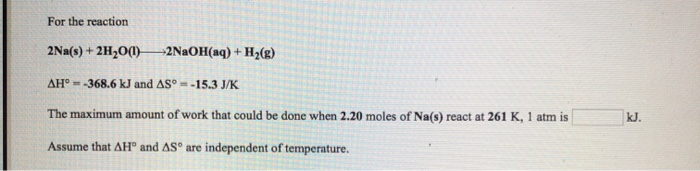We need at least 10 more requests to produce the answer.

0 / 10 have requested this problem solution

The more requests, the faster the answer.

All students who have requested the answer will be notified once they are available.

#### Earn Coins

Coins can be redeemed for fabulous gifts.

Similar Homework Help Questions
• ### Enter your answer in the provided box. Consider the reaction 2Na(s) + 2H2O(1) 2NaOH(aq) + H2)...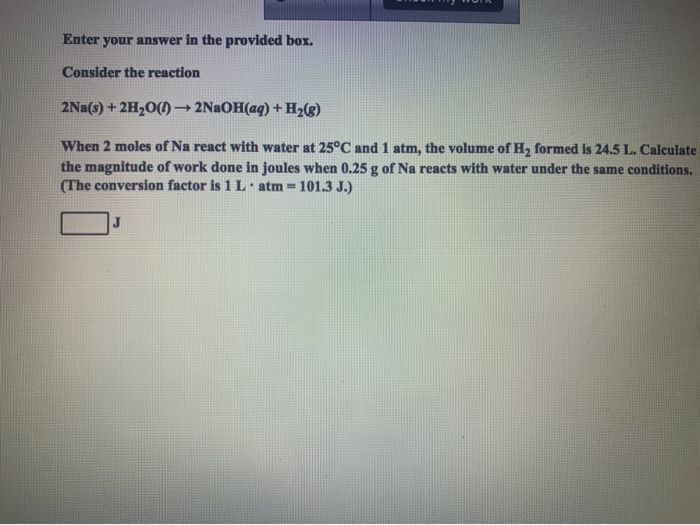Enter your answer in the provided box. Consider the reaction 2Na(s) + 2H2O(1) 2NaOH(aq) + H2) When 2 moles of Na react with water at 25°C and 1 atm, the volume of H2 formed is 24.5 L. Calculate the magnitude of work done in joules when 0.25 g of Na reacts with water under the same conditions. (The conversion factor is 1 L atm = 101.3 J.) J

• ### For the reaction Ca(OH)2(aq) + 2HCl(aq) +CaCl2(s) + 2H2O(1) AH° = -30.2 kJ and AS° =...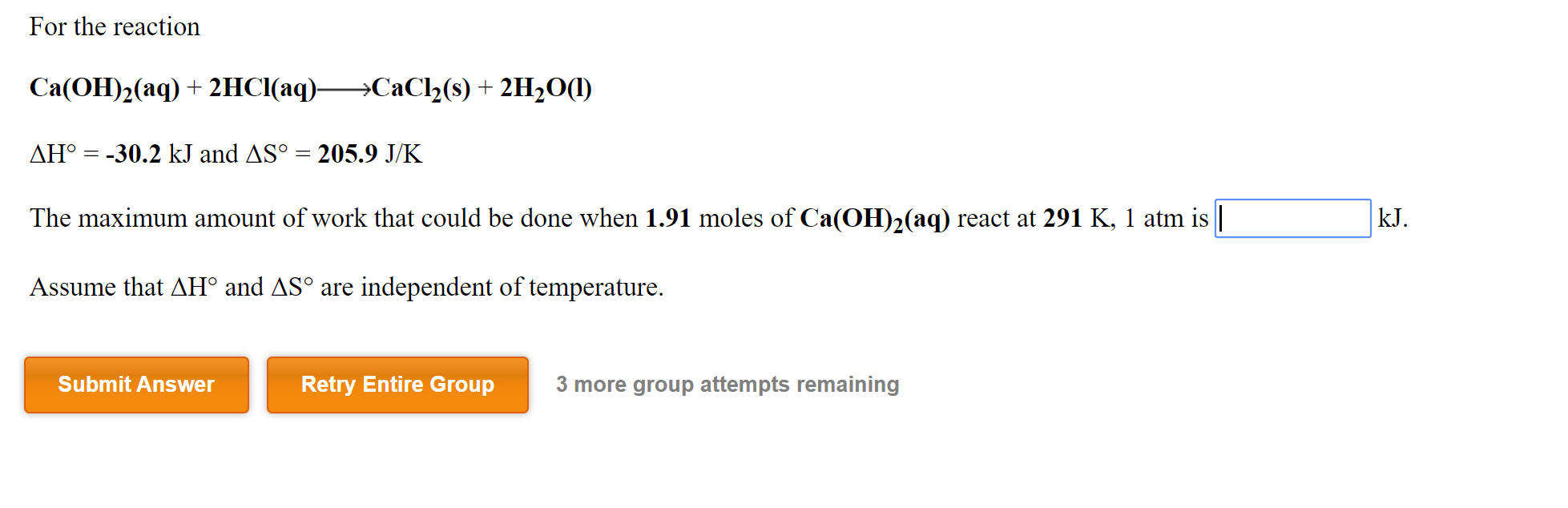For the reaction Ca(OH)2(aq) + 2HCl(aq) +CaCl2(s) + 2H2O(1) AH° = -30.2 kJ and AS° = 205.9 J/K The maximum amount of work that could be done when 1.91 moles of Ca(OH)2(aq) react at 291 K, 1 atm is kJ. Assume that AH° and AS° are independent of temperature. Submit Answer Retry Entire Group 3 more group attempts remaining

• ### For the following reaction: 2Na(s) + 2H2O(l) → 2NaOH(s) + H2(g) Compound ΔH°f (kJ mol-1) S°...

For the following reaction: 2Na(s) + 2H2O(l) → 2NaOH(s) + H2(g) Compound ΔH°f (kJ mol-1) S° (J mol-1 K-1) Na (s) 0.00 51.30 H2O (l) -285.83 69.91 NaOH (s) -425.93 64.46 H2 (g) 0.00 130.68 Calculate ΔG°rx (in kJ) at 391.96 K for this reaction.  Assume ΔH°f and S° do not vary as a function of temperature.

• ### For the reaction He(g)+ CH C;He(e) AG--100.1 kJ and AS--120.7 J/K at 306 K and 1...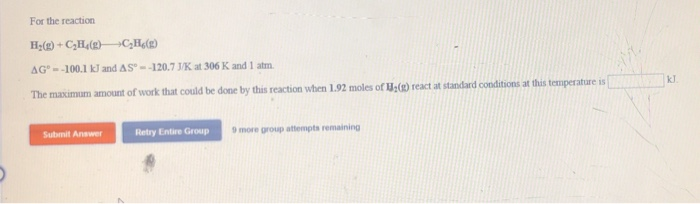For the reaction He(g)+ CH C;He(e) AG--100.1 kJ and AS--120.7 J/K at 306 K and 1 atm. kJ The maximum amount of work that could be done by this reaction when 1.92 moles of He(g) react at standard conditions at this temperature is Submit Answer Retry Entire Group 9 more group attempts remaining For the reaction FeCl; (s)+H2(e) Fe(s)+2HCI(aq) AH--7.4 kJ and AS- 107,9 1/K The maximum amount of work that could be done when 2.12 moles of Fe(s) react...

• ### 1. 2. For the reaction C(S,graphite) +O2(g)CO2(g) 394.4 kJ and AS° = 2.9 J/K at 327...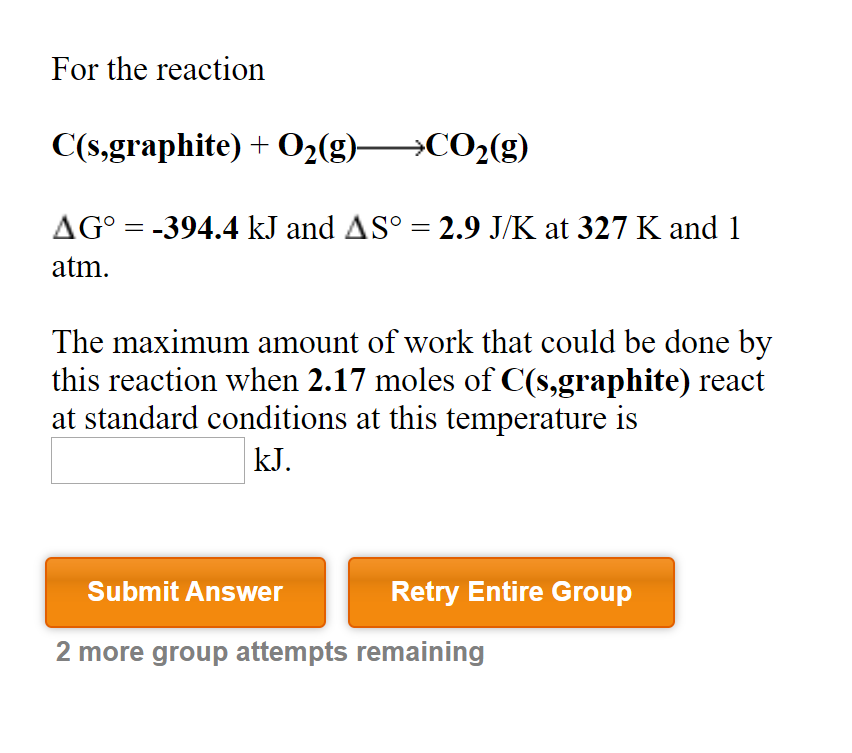1. 2. For the reaction C(S,graphite) +O2(g)CO2(g) 394.4 kJ and AS° = 2.9 J/K at 327 K and 1 AGo atm The maximum amount of work that could be done by this reaction when 2.17 moles of C(s,graphite) react at standard conditions at this temperature is kJ. Submit Answer Retry Entire Group 2 more group attempts remaining For the reaction Ca(OH)2(aq) 2HCI (aq)CaCl2(s)+2H20() = 205.9 J/K AHo-30.2 kJ and ASo The maximum amount of work that could be done when...

• ### Consider this reaction: 2NaOH(aq) + H2(g) →2Na(s) + 2H2O(l)                     Δ H°sys = +367.58 kJ/mol    ΔS°sys = –11.40 J/K·mo

Consider this reaction: 2NaOH(aq) + H2(g) →2Na(s) + 2H2O(l)                     Δ H°sys = +367.58 kJ/mol    ΔS°sys = –11.40 J/K·mol Calculate  ΔSuniv for this reaction at 25 °C

• ### For the reaction Fe(s) + 2HCI(aq)FeC2(8) + H2(2) Δ11°--7.4 kJ and AS。 107.9 J/K The maximum...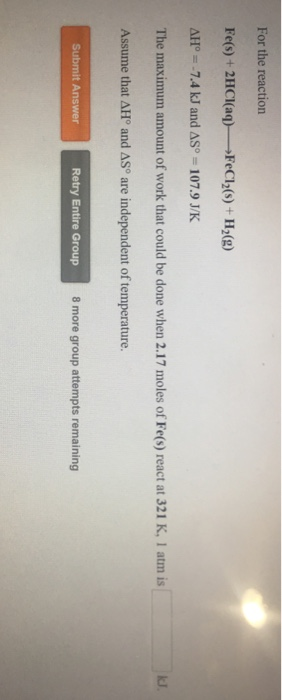For the reaction Fe(s) + 2HCI(aq)FeC2(8) + H2(2) Δ11°--7.4 kJ and AS。 107.9 J/K The maximum amount of work that could be done when 2.17 moles of Fe(s) react at 321 K, 1 atm is Assume that ΔH° and ΔSo are independent of temperature. Submit Answer Retry Entire Group 8 more group attempts remaining

• ### For the reaction 2H2(g) + 302() 2H2O(g) +2502(9) AH° = -1036.0 kJ and AS™ = -152.9...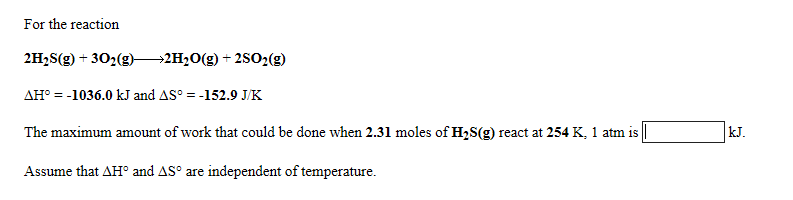For the reaction 2H2(g) + 302() 2H2O(g) +2502(9) AH° = -1036.0 kJ and AS™ = -152.9 JK The maximum amount of work that could be done when 2.31 moles of H2S(g) react at 254 K, 1 atm is Assume that AHⓇ and AS™ are independent of temperature.

• ### Sodium reacts with water according to the reaction: 2Na(s)+2H2O(l)→2NaOH(aq)+H2(g) Identify the oxidizing agent. a) H2O(l) b)...

Sodium reacts with water according to the reaction: 2Na(s)+2H2O(l)→2NaOH(aq)+H2(g) Identify the oxidizing agent. a) H2O(l) b) NaOH(aq) c) Na(s) d) H2 (g)

• ### For the reaction 2CO(g) + O2(g) +2C02(g) AG° = -515.1 kJ and AS = -173.1 J/K...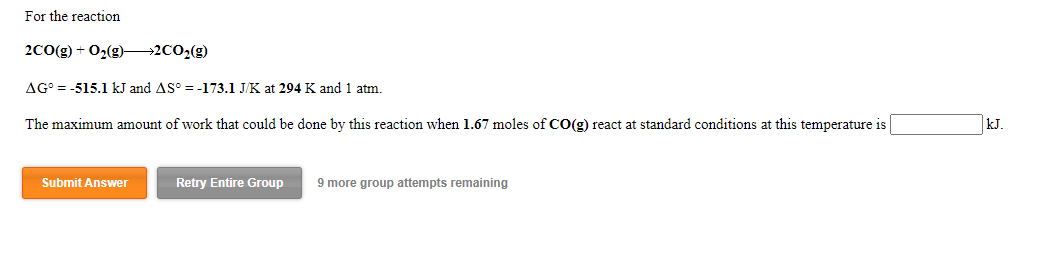For the reaction 2CO(g) + O2(g) +2C02(g) AG° = -515.1 kJ and AS = -173.1 J/K at 294 K and 1 atm. The maximum amount of work that could be done by this reaction when 1.67 moles of CO(g) react at standard conditions at this temperature is kJ Submit Answer Retry Entire Group 9 more group attempts remaining For the reaction CO(g) + 3H2()CH_(g) +H2O(g) AH° = -206.1 kJ and AS° = -214.7 J/K The maximum amount of work that...